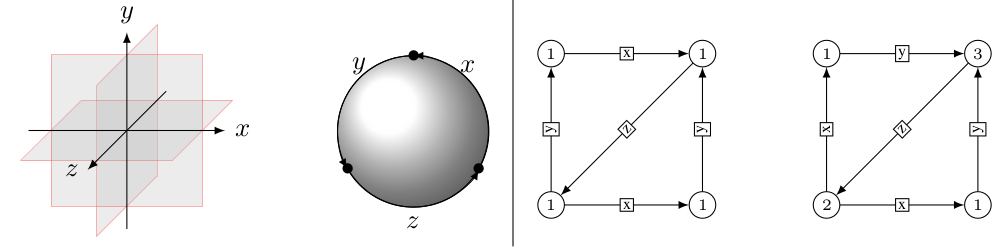# Mirror Symmetry## Course description

Mirror Symmetry investigates a strange connection between two types of geometry: symplectic geometry and algebraic geometry. It was originally discovered in theoretical physics as a duality between two models of string theory: the A- and the B-model. In the 1990's it also became important in mathematics because it could be used to calculate numbers in geometry that mathematicians had tried to find for many years. Since then it has become a main research topic in geometry, algebra and mathematical physics.

In this course we will explore the basic ideas behind mirror symmetry from the point of view of the homological mirror symmetry conjecture. This conjecture formulates an equivalence between two categories: the Fukaya category of a symplectic manifold and the derived category of coherent sheaves of an algebraic variety. We will introduce the mathematics needed to define these two categories such as homology, A-infinity algebras, Floer theory and derived categories. These concepts will be illustrated by some basic examples coming from surfaces. Finally we will work out the mirror correspondence in detail in the cases of the torus.

## Practicalities

The lectures take place on Tuesdays in the afternoon. More info can be found on datanose.

## Syllabus

The course will consist of seven chapters. Each of these chapters will take about 2 weeks. The students will get notes for each chapter with exercises they have to work out. The notes will appear gradually on this site but additional literature is already available for each chapter.

## Exam

Details about the final exam will be discussed during class.

## Structure of the course

The course will treat the following topics.
1. Motivation from physics
2. Homology and Cohomology
3. The A-infinity Formalism
4. Fukaya Categories
5. Matrix Factorizations and Derived categories of Coherent sheaves.
6. The first example: Elliptic curves.
7. The SYZ-conjecture.
• Finding skyscrapers in the Fukaya category
• Moduli spaces of Special Lagrangians
• Stability conditions